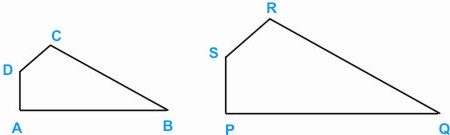HOME MATH DICTIONARY DOWNLOAD FEEDBACK DISCLAIMER
 Question: What is Similar ? Answer: Two objects are similar when they have the sample shape but different size. Paper is introduced in different sizes, A1, A2, A3, A4 and A5, each one being half the size of the one before it, but all the same shape. When a photograph is enlarged to different sizes, all the objects in the photos change size but not shape, so they are similar. Different commodities such as soap powders and breakfast cereals are often sold in cartoons of the same shape but different sizes. Two shapes such as the qudrilateral ABCD and PQRS are similar when the angles which corresponds to one another are equal:∠ A = ∠P; ∠B = ∠Q, ∠C = ∠R, ∠D = ∠S and the ratios of the lengths of the corresponding sides are also equal. In the example shown PQ:AB = QR:BC = RS:CD = SP:DA = 2:1 where the linear scale factor is 2. When two shapes are similar, the ratio of their areas is equal to the square of the ratio of their corresponding lengths, so the area of PQRS is 22 = 4 times the area of ABCD. The enlargement transformation maps any shape onto a similar shape.